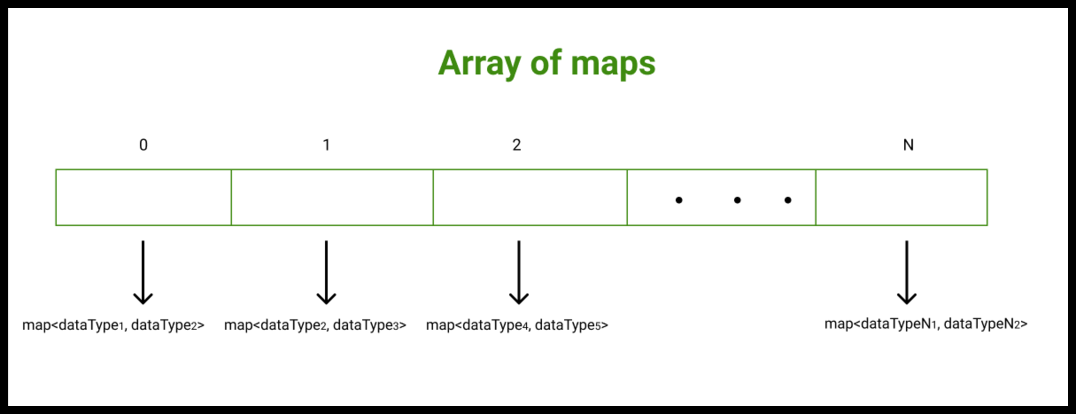# Array of maps in C++ with Examples

• Difficulty Level : Easy
• Last Updated : 27 Dec, 2021

What is an array?

An array in any programming language is a data structure that is used to store elements or data items of similar data types at contiguous memory locations and elements can be accessed randomly using indices of an array. Arrays are efficient when we want to store a large number of elements that too of similar data types.

What is a Map?

In C++, a map is an associative container that is used to store elements in a mapped fashion. Internally, a map is implemented as a self-balancing binary tree. Each element of a map is treated as a pair. The first value is referred to as key and the second value is referred to as value. No two values can have the same key.

Functions associated with Map:

• begin(): Returns an iterator to the first element in the map
• end(): Returns an iterator to the theoretical element that follows the last element in the map
• size(): Returns the number of elements in the map
• max_size(): Returns the maximum number of elements that the map can hold
• empty(): Returns whether the map is empty
• insert(key, Value): Adds a new element to the map
• erase(iterator position): Removes the element at the position pointed by the iterator
• erase(const x): Removes the key-value ‘x’ from the map
• clear(): Removes all the elements from the map

Array of Maps

C++ allows us a facility to create an array of maps. An array of maps is an array in which each element is a map on its own.

Syntax:

map<<dataType1, dataType2>> myContainer[N];

Here,

N: The size of the array of maps
dataType1: The dataType for the key
dataType2: The dataType for the valueExample 1: Below is the C++ program to implement an array of maps.

## C++

 `// C++ program to demonstrate the``// working of array of maps in C++``#include ``using` `namespace` `std;`` ` `// Function to print map elements``// specified at the index, "index"``void` `print(map<``int``, ``bool``>& myMap,``           ``int` `index)``{``    ``cout << ``"The map elements stored at the index "` `<< ``             ``index << ``": \n"``;``    ``cout << ``"Key      Value\n"``;`` ` `    ``// Each element of the map is a pair ``    ``// on its own``    ``for` `(``auto` `pr : myMap) ``    ``{``        ``// Each element of the map is a pair ``        ``// on its own``        ``cout << pr.first << ``"         "` `<< ``                ``pr.second << ``'\n'``;``    ``}`` ` `    ``cout << ``'\n'``;``}`` ` `// Function to iterate over all the array``void` `print(map<``int``, ``bool``>* myContainer, ``int` `n)``{``    ``// Iterating over myContainer elements``    ``// Each element is a map on its own``    ``for` `(``int` `i = 0; i < n; i++) ``    ``{``        ``print(myContainer[i], i);``    ``}``}`` ` `// Driver code``int` `main()``{``    ``// Declaring an array of maps``    ``// In map Key is of type int``    ``// Value is of type bool``    ``map<``int``, ``bool``> myContainer;`` ` `    ``// Mapping values to the map stored``    ``// at the index 0``    ``myContainer = ``true``;``    ``myContainer = ``false``;``    ``myContainer = ``true``;``    ``myContainer = ``false``;`` ` `    ``// Mapping values to the map stored``    ``// at the index 1``    ``myContainer = ``true``;``    ``myContainer = ``false``;``    ``myContainer = ``true``;``    ``myContainer = ``false``;`` ` `    ``// Mapping values to the map stored``    ``// at the index 2``    ``myContainer = ``true``;``    ``myContainer = ``false``;``    ``myContainer = ``true``;``    ``myContainer = ``false``;`` ` `    ``// Calling print function to iterate``    ``// over myContainer elements``    ``print(myContainer, 3);`` ` `    ``return` `0;``}`
Output:
```The map elements stored at the index 0:
Key      Value
10         1
15         0
20         1
25         0

The map elements stored at the index 1:
Key      Value
30         1
35         0
40         1
45         0

The map elements stored at the index 2:
Key      Value
50         1
55         0
60         1
65         0
```

Example 2: Below is the C++ program to implement array of maps.

## C++

 `// C++ program to demonstrate the``// working of array of maps in C++`` ` `#include ``using` `namespace` `std;`` ` `// Function to print map elements specified ``// at the index, "index"``void` `print(map& myMap, ``           ``int` `index)``{``    ``cout << ``"The map elements stored at the index "` `<< ``             ``index << ``": \n"``;``    ``cout << ``"Key      Value\n"``;`` ` `    ``// Each element of the map is a pair ``    ``// on its own``    ``for` `(``auto` `pr : myMap) ``    ``{``        ``cout << pr.first << ``"      "` `<< ``                ``pr.second << ``'\n'``;``    ``}`` ` `    ``cout << ``'\n'``;``}`` ` `// Function to iterate over the map ``// corresponding to an index``void` `print(map* myContainer, ``           ``int` `n)``{``    ``for` `(``int` `i = 0; i < n; i++) ``    ``{``        ``print(myContainer[i], i);``    ``}``}`` ` `// Driver code``int` `main()``{``    ``// Declaring an array of maps``    ``// In map Key is of type string``    ``// Value is of type bool``    ``map myContainer;`` ` `    ``// Mapping values to the map stored ``    ``// at the index 0``    ``myContainer[``"Code"``] = ``true``;``    ``myContainer[``"HTML"``] = ``false``;``    ``myContainer[``"Java"``] = ``true``;``    ``myContainer[``"Solo"``] = ``false``;`` ` `    ``// Mapping values to the map stored ``    ``// at the index 1``    ``myContainer[``"PHP"``] = ``true``;``    ``myContainer[``"CSS"``] = ``false``;``    ``myContainer[``"C++"``] = ``true``;``    ``myContainer[``"Lab"``] = ``false``;`` ` `    ``// Mapping values to the map stored ``    ``// at the index 2``    ``myContainer[``"Swift"``] = ``true``;``    ``myContainer[``"Cobol"``] = ``false``;``    ``myContainer[``"Fizzy"``] = ``true``;``    ``myContainer[``"Pizza"``] = ``false``;`` ` `    ``// Calling print function to print``    ``// myContainer elements``    ``print(myContainer, 3);`` ` `    ``return` `0;``}`
Output:
```The map elements stored at the index 0:
Key      Value
Code      1
HTML      0
Java      1
Solo      0

The map elements stored at the index 1:
Key      Value
C++      1
CSS      0
Lab      0
PHP      1

The map elements stored at the index 2:
Key      Value
Cobol      0
Fizzy      1
Pizza      0
Swift      1
```

My Personal Notes arrow_drop_up